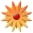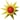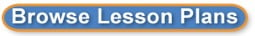# Integrating Technology for At-Risk Learners for the Pythagorean Theorem

Page Views: 3758

Email This Lesson Plan to Me
Overall Rating:(5.0 stars, 1 ratings)

 Subject(s): Math Grade 8 NETS-S Standard: Communication and CollaborationCritical Thinking, Problem Solving, and Decision MakingTechnology Operations and ConceptsView Full Text of Standards School: Wesley International Academy, Atlanta, GA Planned By: Rachel Goodman Original Author: Rachel Goodman, Atlanta
Essential Question:
How do you find the length of the hypotenuse given the lengths of both legs of a right triangle?

CCGPS Standard:
MCC8.G.6. Explain a proof of the Pythagorean Theorem and its converse.
MCC8.G.7. Apply the Pythagorean Theorem to determine unknown side lengths in right triangles in real-world and mathematical problems in two and three dimensions.

Objective:
Given a worksheet, the student will find the missing hypotenuse length of a right triangle using the Pythagorean Theorem correctly 7 out of 10 times.

Procedures
Introduction
1. Anticipatory Set
The teacher will begin the lesson by stating, Today we are going to continue our study of exponents and square roots by applying them to something called the Pythagorean Theorem. A famous mathematician named Pythagoras discovered the Pythagorean Theorem a long time ago. The Pythagorean Theorem applies to a very specific triangle, a Right Triangle and by the end of this lesson you are going to use what Pythagoras discovered many years ago to find missing side lengths of a right triangle. The teacher will utilize the first form of technology using Padlet. On the student iPads, students will sign in to padlet.com at the teacher will display the padlet entitled, What We Know About Right Triangles on the Promethean Board. The teacher will give students five minutes to type and post something about right triangles on the class padlet. Student responses will generate on the padlet posted on the Promethean Board. Teacher may want to prompt students some ideas such as characteristics, where they see them in the real world, etc. Once time is completed, the teacher will spend three minutes discussing some responses that were posted.
2. Purpose in students life
After teacher reads some student examples, the teacher will say,  As you can see, right triangles are unique types of triangles and serve multiple purposes in our study of mathematics and geometry. Today, you will quickly find out that the Pythagorean Theorem will become very useful when dealing with side lengths of right triangles. The Pythagorean Theorem can be used to classify the size of TVs, determine the length of a shadow, and see how tall a tent stands! The Pythagorean Theorem is used in all sorts of real world scenarios.

Implementation
Input

On the Promethean Board, the teacher will pull up the PowerPoint entitled, The Pythagorean Theorem. The students will be told the formal definition of the Pythagorean Theorem: A theorem stating that the square of the length of the hypotenuse (the longest side) of a right triangle is equal to the sum of the squares of the lengths of the other sides. It is mathematically stated as c2 = a2 + b2, where c is the length of the hypotenuse and a and b the lengths of the other two sides. The teacher will state, Because of the Pythagorean Theorem, we can find the lengths of a missing side of a right triangle. Once again, it is very important for us to use the new vocabulary you learned today correctly- leg and hypotenuse. The legs, a and b, can be used interchangeably. However, the hypotenuse, longest side, must always be represented by the letter c. It is also important to note that the hypotenuse is always the side across from the right angle. Allow students to write the definition and formula in their notes. The teacher will then write the formula and a right triangle largely on the board as the students copy the diagram into their notes. The teacher will then draw arrows from the formula to the triangle to the vocabulary words allowing the students to see the legs of a triangle and the hypotenuse and how they are represented in the formula. The teacher will say, Now I am going to show you how to use the Pythagorean Theorem to find the length of the hypotenuse given the lengths of the legs. The teacher will draw a right triangle with side lengths of 3 cm and 4 cm on the board. The teacher will say, Now, I have a right triangle with side lengths of 3 cm and 4 cm. However, I notice that the hypotenuse length is missing. Since the hypotenuse length is unknown, I will use the variable c to represent it. According to our notes, we found that . I am now going to substitute the values into the formula to find the length of the hypotenuse, or c. The teacher shows the next step and writes on the board. The teacher will say, According to Order of Operations, I must perform the exponents first. Therefore, I end up with 9 + 16 = c2. I know that 9 + 16 is 25 so 25 = c2. A few lessons ago, we learned that taking the square root of a number is the inverse operation of squaring a number. Therefore, in order to isolate the variable, c, by itself, I must take the square root of each side. Therefore, c=5. I can now state that by using the Pythagorean Theorem, the length of the hypotenuse is 5 cm long. The teacher will pause in modeling the steps to using the Pythagorean Theorem to find missing side lengths in order to allow students to take notes, to review the steps in the process, and to answer any student questions in the initial phase of checking for understanding. After the pause, the teacher will choose to construct two more examples on the board with side lengths- 6 cm and 8 cm and 9 ft and 12 ft and model how to solve for the missing hypotenuse length.

Modeling/Examples
The teacher started the lesson by modeling how to construct Triangle ABC on the GeoGebra App. The teacher also modeled where the legs and hypotenuse were located both in a right triangle as well as the formula for the Pythagorean Theorem. The teacher modeled through the initial example of a triangle with side lengths of 3 cm and 4 cm. It was very important for the teacher to show the following steps while using the Pythagorean Theorem formula:
1. Representing the hypotenuse as c
2. Substituting the values in for legs a and b.
3. Performing order of operations for exponent values.
4. Combining like terms by adding the squares of the legs.
5. Performing inverse operations to isolate the variable c and find the length of the hypotenuse.
The teacher may choose to perform more examples on the board with different leg lengths in a right triangle. The most important aspect of the teacher modeling is to show the step-by-step process as well as model think-alouds in order to ensure the proper use of vocabulary.

Checking for Understanding
At this point, the teacher will ask students to take out their smartphones and login to Kahoot! Using the login pin provided, students will login to Kahoot! to answer three questions regarding the Pythagorean Theorem. The teacher will say, Lets see if we have any remaining questions before we move into guided practice. This quick check will allow me to see what I may need to go back and reteach or review regarding the Pythagorean Theorem. Kahoot! is a connected learning platform that allows the teacher to create a brief quiz that students will answer on their smartphone. After each question, it shows the teacher and students how many correct responses there were and what incorrect responses were chosen. This particular Kahoot! will consist of three questions:
1. A question that will have students identify the legs and hypotenuse
2. A question that will have students identify the formula for Pythagorean Theorem
3. A question that will have students find the missing hypotenuse of a right triangle.
After the small check for understanding, the teacher will have insight into what misunderstandings students may have regarding the information they just learned. The teacher will go back and address those issues by reteaching or explaining those concepts.
Guided Practice
Once students have shown a general understanding of how to use the Pythagorean Theorem, the teacher will say, Now that you have watched me work through applying the Pythagorean Theorem to find the side lengths of a hypotenuse. It is your turn to try. I will give you a right triangle whose leg lengths are known but whose hypotenuse is missing. Lets take out our GeoGebra app on the iPad so we can first construct this triangle. I want you to construct a triangle with leg lengths of 5 cm and 12 cm. In order for us to apply the Pythagorean Theorem, it must be what kind of triangle? Allow for a student response and allow time for students to construct the right triangle on their iPad. The teacher should be actively walking around the classroom to identify students who are still confusing the legs and hypotenuse. Once everyone has correctly labeled the legs and not the hypotenuse the teacher will say, Now that we have correctly identified the legs with lengths of 5 cm and 12 cm. Lets apply the Pythagorean Theorem to see if we can find the length of the hypotenuse. What is the formula I will use? Allow for student responses and have students work through the problem in their notebook. The teacher will actively monitor student responses by walking around the room. It is imperative that the students show each step in the process so that the teacher may identify where students are making errors. The teacher will then say, Most of you have arrived at 13 cm as the length of the hypotenuse. Using the measuring tool on GeoGebra, check your answer to see if it makes sense. Students will check their answer and they should have a length of 13 cm.

Independent Practice
The teacher will say, Now I want to see what you are able to do without the help of GeoGebra so please return your iPads to the cart and find your seat. I will now pass out a worksheet that you will complete independently. The worksheet consists of ten problems in which you are to find the length of the hypotenuse. Remember in order to do that we must apply the formula for the Pythagorean Theorem. As you are completing this worksheet, I want you to show each step of your process. The teacher will pass out the worksheet and have students complete the problems. The worksheet consists of ten problems where students will be required to apply the Pythagorean Theorem to find the length of the hypotenuse. The teacher will collect this independent practice and use it to evaluate student mastery of the objective.

Closure
The teacher will say, Today we learned how to find the length of a hypotenuse using the Pythagorean Theorem. Remember, the theorem states that in a right triangle, . We must always represent the legs using the variables a and b and the hypotenuse using the variable c. We can easily identify the hypotenuse because it is the longest side of a right triangle and across from the right angle. We also were able to apply our knowledge of exponents and square roots while working with the formula. Once we substitute and simplify our formula, we must take the square root at the end in order to perform the inverse operation and get c alone. Tomorrow, we are going to learn what to do when we have the length of the hypotenuse and one leg and need to find the length of the other leg. We will use GeoGebra to find the similarities and differences between the problems we had today and what we will learn tomorrow.

Materials
GeoGebra App
Promethean Board
GeoGebra Student Chart
PowerPoint
Smartphones
Kahoot! Quiz
Calculators
Independent Practice Worksheet
Student Notebooks
Remediation materials if needed: student laptop, headphones, whiteboard, marker, and remediation examples worksheet.
Enrichment materials if needed: Three Pythagorean Theorem proofs activity

Evaluation
The teacher will evaluate student mastery of the objective by collecting the independent practice worksheet. Students will be required to find the hypotenuse length of a right triangle correctly 7 out of 10 times. In addition to the formal evaluation, the teacher will conduct informal evaluations by observing behavior throughout the lesson and guided practice.

Remediation:
Students who have still not mastered the concept during the lesson will be provided with a pair of headphones and student laptop. The students will be given a video tutorial on the Pythagorean Theorem in which the teacher breaks down each step slowly and carefully. The student will be given a white board and marker to work through additional examples after the tutorial has been completed.

Enrichment:
For those students who have quickly grasped the concept, an activity will be given to them regarding the three different ways to prove the Pythagorean Theorem. These three proofs are those done by Pythagoras himself, President Garfield, and the Hindu mathematician Bhaskara. The activity will have the students work through step-by-step of how each mathematician proved the Pythagorean Theorem and have students generalize the similarities and differences between each of the proofs. Students should make generalized statements regarding which one makes most sense to them and how it connects to what they learned in todays lesson in their math notebook.
 Cross-Curriculum Ideas Physical Science- Vectors Follow-Up See enrichment section in lesson plan Materials: Whiteboards, Middle Other Items: 25 iPads, \$299.00 each, total of \$7475.00